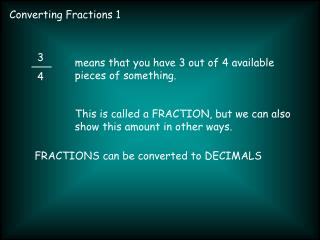DownloadDownload PresentationConverting Fractions 1

# Converting Fractions 1

Télécharger la présentation## Converting Fractions 1

- - - - - - - - - - - - - - - - - - - - - - - - - - - E N D - - - - - - - - - - - - - - - - - - - - - - - - - - -
##### Presentation Transcript

1. Converting Fractions 1 3 4 means that you have 3 out of 4 available pieces of something. This is called a FRACTION, but we can also show this amount in other ways. FRACTIONS can be converted to DECIMALS

2. Converting Fractions 1 - DECIMALS DECIMALS aren’t as accurate as fractions, but are sometimes easier to understand. 1 25 of £1 isn’t as easy to understand as £0.04 (or 4p) Decimals are easier to put in order than fractions, especially with a calculator.

3. Converting Fractions 1 - DECIMALS To convert any FRACTION to a DECIMAL with a calculator we just type: NUMERATOR ÷ DENOMINATOR = (top number) (bottom number) 1 25 becomes 1 ÷ 25 = 0.04

4. Converting Fractions 1 - DECIMALS To convert any FRACTION to a DECIMAL without a calculator we have to do a DIVISION calculation: 1 ÷ 25 25 ) 1

5. Converting Fractions 1 - DECIMALS 1 shared into 25 parts can’t go, so just add a 0 after the DECIMAL POINT 0 . 1 ÷ 25 25 ) 1 . 0 pretend that the decimal point isn’t there, and read this as 10 instead of 1.0

6. Converting Fractions 1 - DECIMALS 10 shared into 25 parts can’t go, so we add another 0 0 . 0 1 ÷ 25 25 ) 1 . 0 0

7. Converting Fractions 1 - DECIMALS 100 shared into 25 parts goes exactly with no remainder 0 . 0 4 1 ÷ 25 25 ) 1 . 0 0

8. Converting Fractions 1 - DECIMALS sometimes we have a remainder. 0 1 ÷ 3 3 ) 1

9. Converting Fractions 1 - DECIMALS 10 ÷ 3 is 3 with 1 remaining 0 . 3 1 ÷ 3 3 ) 1 . 0 1

10. Converting Fractions 1 - DECIMALS 10 ÷ 3 is 3 with 1 remaining 0 . 3 3 1 ÷ 3 3 ) 1 . 0 0 1 1

11. Converting Fractions 1 - DECIMALS 10 ÷ 3 is 3 with 1 remaining 0 . 3 3 3 1 ÷ 3 3 ) 1 . 0 0 0 1 1 1 once we can see a pattern we can see what the next numbers will be.

12. Converting Fractions 1 - DECIMALS 10 ÷ 3 is 3 with 1 remaining 1 ÷ 3 = 0.33333333333333…… . this can be written as 0 . 3 read as 0 . 3 RECURRING The dot means repeat this number.